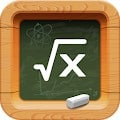# Math Tests APK

Math for primary and secondary school students.Improve your math fluency through dozens of multiple-choice tests. You will receive a grade at the end of each test.

-addition and subtraction up to 100
-basic geometry 2D/3D figures and symmetry

-multiplication and division
-ten based system and place value (expanded form of a number, value of digits)
-metric units of measurements (length, weight, volume, area – meters, km, liters, tons)
-US standard units of measures (cups-c, pints-pt, fl oz, yards-yd,inches-in, feet-ft,ounces, pounds-lb)
-time (minutes, hours, seconds, days, weeks), converting am/pm time to 24 hour time

-long subtraction and addition (in columns with or without carrying), multiplication, division, divisibility, operations with brackets)
-rounding
-Roman numerals up to 1 000 and Greek alphabet
-ten based system and place value (expanded form, value of digits)

-fraction practice (numerator and denominator, all operations, compare with whole numbers, mixed numbers, complex fractions, fractions in the lowest term, adding and subtracting fractions, multiplying fractions, dividing fractions)
-rounding to the nearest ten, hundred or thousand
-decimals (decimals and fractions, compare with fractions, multiplying and dividing decimals)

App also includes:
-divisibility (odd and even numbers, divisibility by 3, 4, 5, 6, 8, 9, 10)
-negative numbers and absolute value (compare negative numbers, add and subtract negative numbers, multiplying and dividing negative numbers, adding and subtracting absolute value)
-equations with variables (one-step equations, equations with negative numbers, quadratic equations)
-inequalities and intervals (inequalities with all operations and negative numbers)
-geometry (number of sides, faces and vertices, area of squares, triangles, trapezoids and rhomboids, altitudes and medians, volume of prisms, pyramids, cones, spheres, perimeters)
-square roots, powers and exponents (negative base, negative exponent, fractions as exponents, numerical and algebraic expressions with exponents, scientific notation)
-basic algebra
-sets, set operations, Venn diagrams and types of numbers
-percents %, ratios, cross-multiplication word problems, the rule of three, direct and indirect variation
-angles and their types (right, obtuse, acute; adjacent, vertical, linear pair, alternate, corresponding, consecutive), angles at the center and circumference
-linear functions, range and domain

More will be added soon since our aim is to cover complete curriculum for Primary and High school. This app is suitable not only for children but also for older students and adults.

Results are tracked. You can review your mistakes and progress. The app works offline as well!

This app is like having dozens of math worksheets and exercises for 1st, 2nd, 3rd, 4th, 5th, 6th and 7th grade/class directly in your device with results and solutions immediately available. Since it works offline you learn to solve math and algebra problems whenever you want! The exercises are ideal for better math fluency and are suitable for kids and adults as well.

Key features overview:
• dozens of math categories and subcategories
• thousands of questions
• each test is evaluated – you are given a grade like in school
• test history allows you to see where you made mistakes
• statistics showing your progress over time
• includes a math championship where you can compete with other users to learn math in fun way

#### What’s New

– New feature Parent connect – watch children learning progress on parent phone or tablet.

Name
Math Tests
Package
com.holucent.math
Version
1.4.8
Size
16.74 MB
Installs
Developed By
Holucent

• Knights says:

This site is great 😀 thanks

• Nic Andrew says:

Great website! thanks

• McShiddie Gryffindor says:

One of the best site for android apps. i appreciate it so much ! so much fun !

• iZONEGIGABYTE says:

Thanks for This site, it very enjoy 🙂

• pandidurai says:

vry vry usefull site realy i like tis

• wilfredo says:

it is really great thanks for dis !

• Ali k. says:

very good website.
I really enjoyed very.

This site uses Akismet to reduce spam. Learn how your comment data is processed.